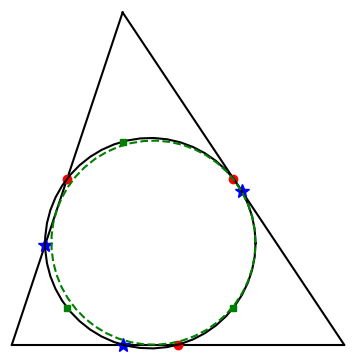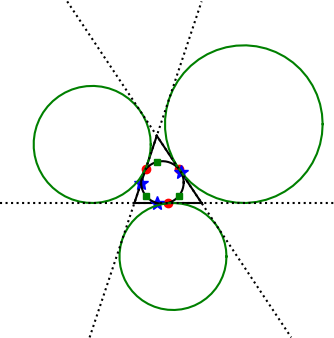# Incircle and excircles

An earlier post looked at the nine-point circle of a triangle, a circle passing through nine special points associated with a triangle. Feuerbach’s theorem that says the nine point circle of a triangle is tangent to the incircle and three excircles of the same triangle.

The incircle of a triangle is the largest circle that can fit inside the triangle. When we add the incircle to the illustration from the post on the nine-point circle, it’s kinda hard to see the difference between the two circles. The nine-point circle is drawn in solid black and the incircle is drawn in dashed green.If we extend the sides of the triangle, an excircle is a circle tangent to one side the original triangle and to the extensions of the other two sides.